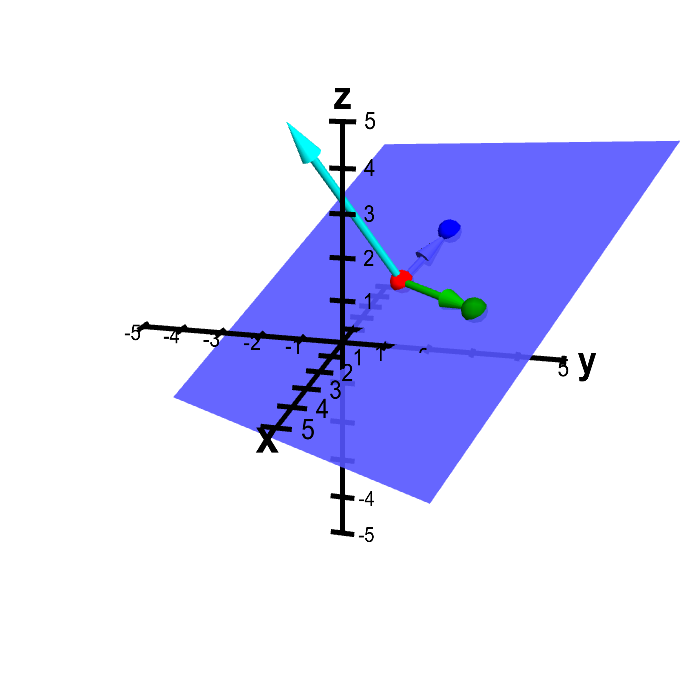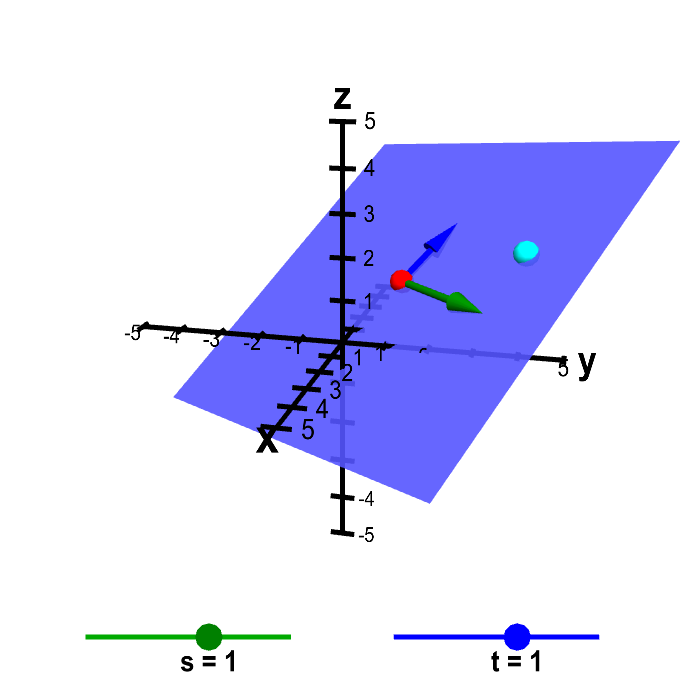# Math Insight

### Parametrization of a plane

A plane is determined by a point $\vc{p}$ in the plane and two vectors $\vc{a}$ and $\vc{b}$ parallel to the plane. (This is identical to the case with three points in the plane. Why?)Plane determined from three points. The plane is determined by the points $\color{red}{P}$ (in red), $\color{green}{Q}$ (in green), and $\color{blue}{R}$ (in blue), which you can move by dragging with the mouse. The vectors from $\color{red}{P}$ to both $\color{green}{Q}$ and $\color{blue}{R}$ are drawn in the corresponding colors. The normal vector (in cyan) is the cross product of the green and blue vectors.

From this fact, we can parametrize a plane just like we parametrized a line (only we'll need two parameters instead of one). If $\vc{x}$ is a point in the plane, the vector from $\vc{p}$ to $\vc{x}$ (i.e., $\vc{x}-\vc{p}$) is some multiple of $\vc{a}$ plus some multiple of $\vc{b}$. (Can you see why?) We can express this as $\vc{x}-\vc{p} = s\vc{a} + t\vc{b}$ for $t,s \in \vc{R}$. Usually, we'll write this as $\vc{x} = \vc{p} + s\vc{a} + t \vc{b}$. The real numbers $s$ and $t$ are the parameters for this parametrization of the line plane.

The idea of the parametrization is that as the parameters $s$ and $t$ sweep through all real numbers, the point $\vc{x}$ sweeps out the plane. In other words, it is a two-dimensional analogue of the parametrization of the line.

Here we've added the point $\vc{x}$ in cyan. You can move $\vc{x}$ directly or by changing the parameters $s$ and $t$ (the points on sliders).Parametrization of a plane. The plane is determined by the point $\color{red}{\vc{p}}$ (in red) and the vectors $\color{green}{\vc{a}}$ (in green) and $\color{blue}{\vc{b}}$ (in blue), which you can move by dragging with the mouse. The point $\vc{x} = \vc{p} + s\vc{a} + t \vc{b}$ (in cyan) sweeps out all points in the plane as the parameters $s$ and $t$ sweep through their values. You can move the cyan point $\vc{x}$ either by changing the values of $s$ and $t$ with the sliders or by moving the point directly (which updates the values of $s$ and $t$). For this applet, the values of $s$ and $t$ are restricted to be between -5 and 5, which restricts the possible locations of the cyan point, depending on the values of $\vc{a}$ and $\vc{b}$.

Clearly, for any value of $s$ and $t$, the point $\vc{x}$ lies on the plane. Also, by changing $s$ and $t$, you can move the point $\vc{x}$ to any position on the plane (subject to the restriction of the applet that keeps $s$ and $t$ between -5 and 5).

You can see an example of parametrizing a plane here.